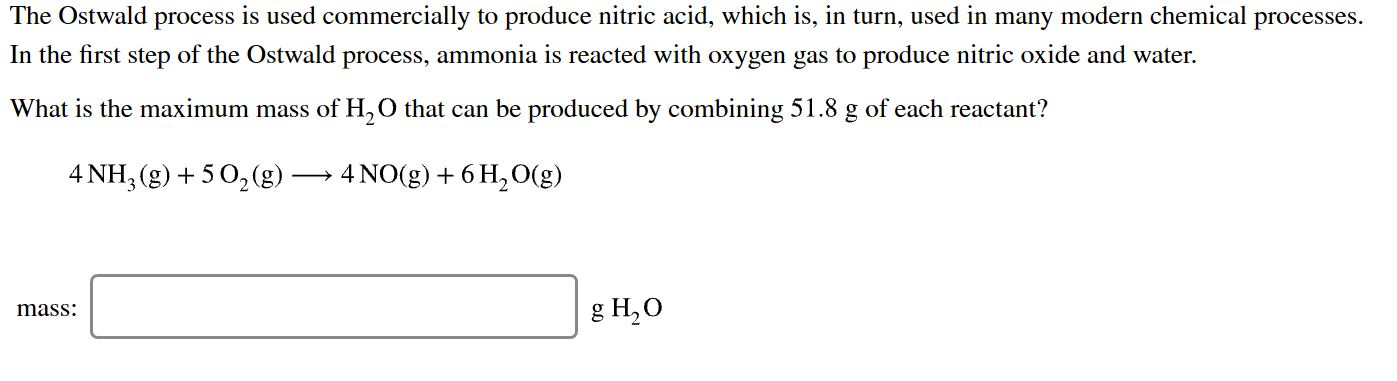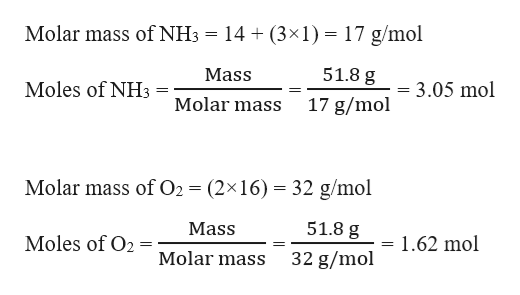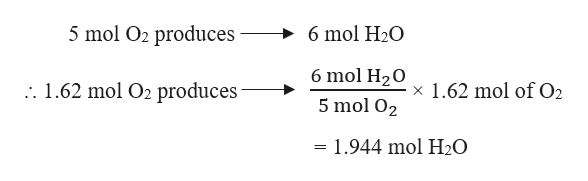# The Ostwald process is used commercially to produce nitric acid, which is, in turn, used in many modern chemical processesIn the first step of the Ostwald process, ammonia is reacted with oxygen gas to produce nitric oxide and waterWhat is the maximum mass of H,O that can be produced by combining 51.8 g of each reactant?4 NH, (g)5 02(g)4 NO (g) 6H2O(g)8 H,Оmass

Question
1 viewshelp_outlineImage TranscriptioncloseThe Ostwald process is used commercially to produce nitric acid, which is, in turn, used in many modern chemical processes In the first step of the Ostwald process, ammonia is reacted with oxygen gas to produce nitric oxide and water What is the maximum mass of H,O that can be produced by combining 51.8 g of each reactant? 4 NH, (g)5 02(g) 4 NO (g) 6H2O(g) 8 H,О mass fullscreen
check_circle

Step 1

Given,

Mass of NH3 = 51. 8 g

Mass of O2 = 51. 8 g

Moles of NH3 and O2 can be calculated as:help_outlineImage TranscriptioncloseMolar mass of NH3 = 14 (3x1) = 17 g/mol 51.8 g Mass Moles of NH3 - 3.05 mol 17 g/mol Molar mass Molar mass of O2 (2x16) 32 g/mol 51.8 g Mass Moles of O2 1.62 mol 32 g/mol Molar mass fullscreen
Step 2

The balanced chemical reaction is given as:

4 NH3 (g) + 5 O2 (g) → 4 NO (g) + 6 H2O (g)

Stoichiometric molar ratio of NH3 : O2 = 4 : 5 = 1 : 1.25

Actual molar ratio of NH3 : O2 = 3.05 : 1.62 = 1 : 0.53

Therefore, NH3 is present in excess and O2 is the limiting reagent. Therefore, the mass of H2O produced will depend on O2.

Step 3

From the given reaction, i...help_outlineImage Transcriptionclose5 mol O2 produces 6 mol H2O 6 mol H20 . 1.62 mol O2 produces x 1.62 mol of O2 5 mol O2 = 1.944 mol H20 fullscreen

### Want to see the full answer?

See Solution

#### Want to see this answer and more?

Solutions are written by subject experts who are available 24/7. Questions are typically answered within 1 hour.*

See Solution
*Response times may vary by subject and question.
Tagged in

### General Chemistry